# Count the number of Keys in a Dictionary in PythonLast updated: Feb 18, 2023
4 min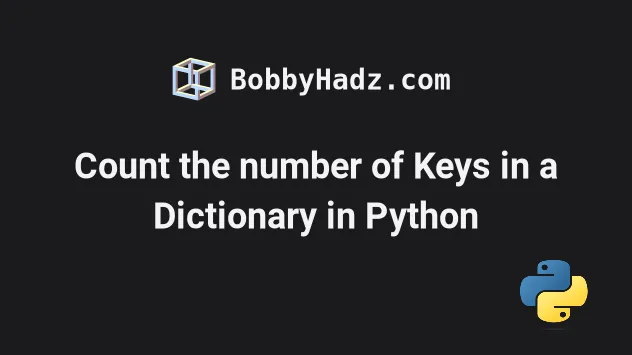## #Count the number of Keys in a Dictionary in Python

Use the `len()` function to count the number of keys in a dictionary, e.g. `result = len(my_dict)`.

The `len()` function can be passed a collection such as a dictionary and will return the number of key-value pairs in the dictionary.

main.py
```Copied!```my_dict = {
'age': 30,
}

result_1 = len(my_dict)
print(result_1)  # 👉️ 3

result_2 = len(my_dict.keys())
print(result_2)  # 👉️ 3
``````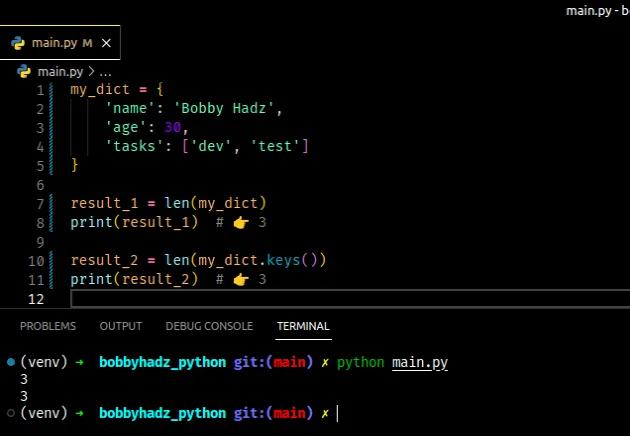The len() function returns the length (the number of items) of an object.

The argument the function takes may be a sequence (a string, tuple, list, range or bytes) or a collection (a dictionary, set, or frozen set).

The first example shows how to get the number of key-value pairs in the dictionary.

main.py
```Copied!```my_dict = {
'age': 30,
}

result_1 = len(my_dict)
print(result_1)  # 👉️ 3
``````
The number of keys and values in the dictionary is the same, so passing the entire dictionary to the `len()` function is sufficient.

## #Explicitly using the `dict.keys()` method

The dict.keys method returns a new view of the dictionary's keys.

main.py
```Copied!```my_dict = {'id': 1,  'name': 'Bobby Hadz'}

print(my_dict.keys())  # 👉️ dict_keys(['id', 'name'])

print(len(my_dict.keys()))  # 👉️ 2
``````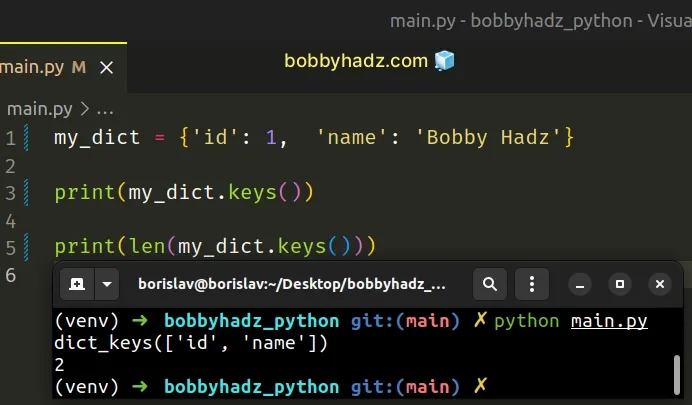You can use the list() class if you need to convert the view of keys into a list.

main.py
```Copied!```my_dict = {'id': 1,  'name': 'Bobby Hadz'}

list_of_keys = list(my_dict.keys())
print(list_of_keys)  # 👉️ ['id', 'name']

print(len(list_of_keys))  # 👉️ 2
``````

Note that passing the dictionary directly to the `len()` function is a little faster than creating a view object via the `dict.keys()` method and passing the view to the `len()` function.

## #Using a for loop to count the number of keys in a dictionary

You can also use a for loop to count the number of keys in a dictionary.

main.py
```Copied!```my_dict = {
'age': 30,
}

count = 0

for key, value in my_dict.items():
count += 1

print(key, value)

print(count) # 👉️ 3
``````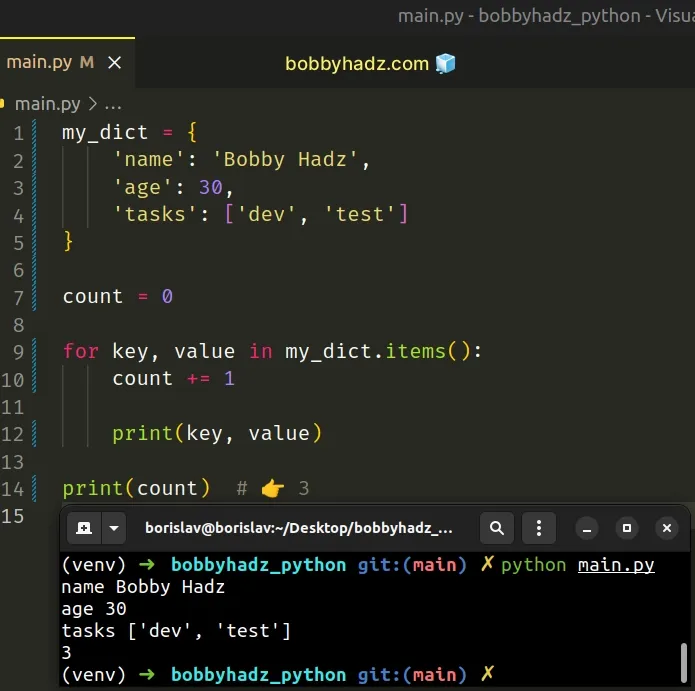We used a `for` loop to iterate over the dictionary's items and on each iteration, we increment the `count` variable.

The dict.items method returns a new view of the dictionary's items ((key, value) pairs).

main.py
```Copied!```my_dict = {'id': 1,  'name': 'BobbyHadz'}

# 👇️ dict_items([('id', 1), ('name', 'BobbyHadz')])
print(my_dict.items())
``````

If you don't need to access the key and value while iterating, iterate over the dictionary directly.

main.py
```Copied!```my_dict = {
'age': 30,
}

count = 0

for key in my_dict:
count += 1

print(key)

print(count)  # 👉️ 3
``````

On each iteration, we get access to the key in the `for` loop and increment the value of the `count` variable.

## #Count the number of dictionary keys with values matching condition

You can also use a `for` loop if you need to count the number of dictionary keys with a particular value.

main.py
```Copied!```my_dict = {
'a': 1,
'b': 10,
'c': 30,
}

count = 0

for key, value in my_dict.items():
if value > 5:
count += 1
print(key, value)

print(count)  # 👉️ 2
``````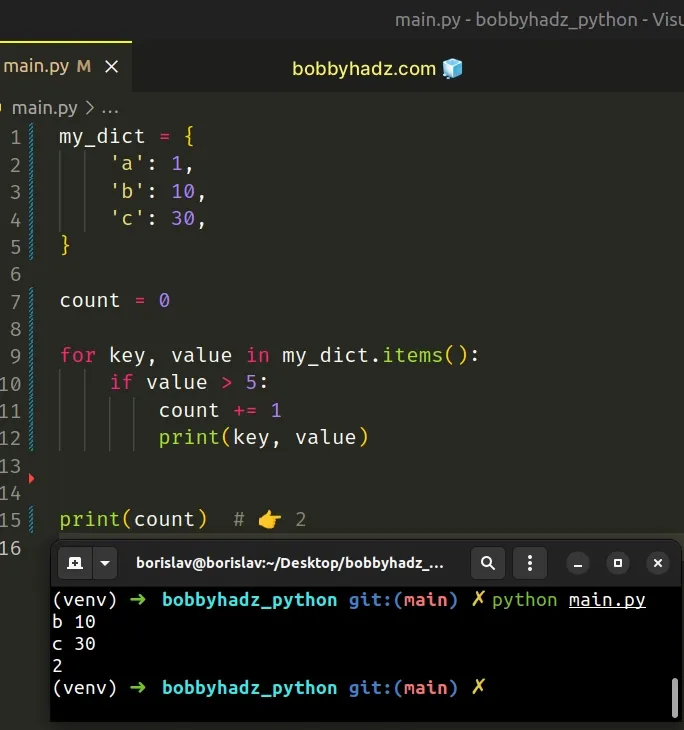We used a `for` loop to iterate over the dictionary's items.

On each iteration, we check if the current value is greater than `5`.

If the condition is met, we increment the `count`.

The dictionary has 2 keys that have values greater than `5`, so the `count` variable stores a value of `2` after the last iteration.

## #Checking if the dictionary is empty

You can also use the `len()` function to check if a dictionary is empty.

main.py
```Copied!```my_dict = {}

if len(my_dict) == 0:
# 👇️ this runs
print('dict is empty')
else:
print('dict is not empty')
``````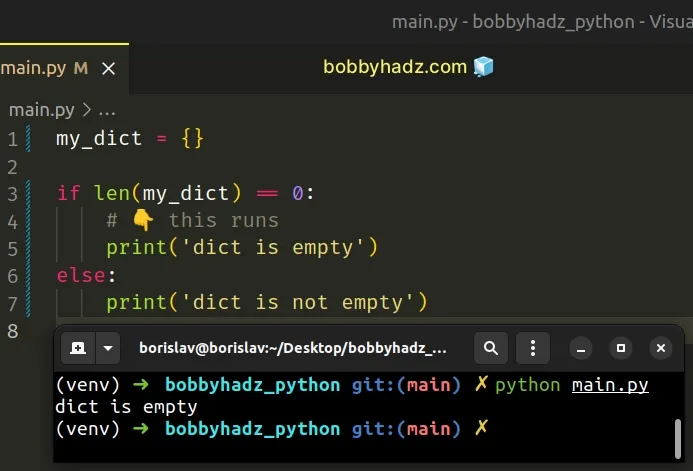If a dictionary has a length of `0`, then it's empty.

You might also see examples online that check whether the dictionary is truthy (to check if it contains at least 1 key-value pair), which is more implicit.

main.py
```Copied!```my_dict = {}

if my_dict:
print('dict is NOT empty')
else:
# 👇️ this runs
print('dict is empty')
``````

All values that are not truthy are considered falsy. The falsy values in Python are:

• constants defined to be falsy: `None` and `False`.
• `0` (zero) of any numeric type
• empty sequences and collections: `""` (empty string), `()` (empty tuple), `[]` (empty list), `{}` (empty dictionary), `set()` (empty set), `range(0)` (empty range).

Notice that an empty dictionary is a falsy value, so if the `dict` is empty, the `else` block is run.

If you need to check if the dictionary is empty using this approach, you would negate the condition with `not`.

main.py
```Copied!```my_dict = {}

if not my_dict:
# 👇️ this runs
print('dict is empty')
else:
print('dict is NOT empty')
``````